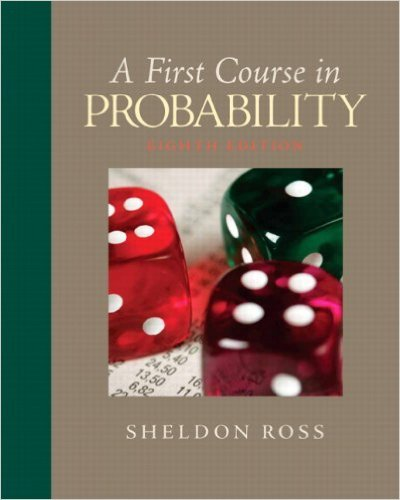×

×

# Solutions for Chapter 10: Simulation## Full solutions for First Course in Probability | 8th Edition

ISBN: 9780136033134Solutions for Chapter 10: Simulation

Solutions for Chapter 10
4 5 0 321 Reviews
17
3
##### ISBN: 9780136033134

Summary of Chapter 10: Simulation

This expansive textbook survival guide covers the following chapters and their solutions. This textbook survival guide was created for the textbook: First Course in Probability, edition: 8. First Course in Probability was written by and is associated to the ISBN: 9780136033134. Chapter 10: Simulation includes 16 full step-by-step solutions. Since 16 problems in chapter 10: Simulation have been answered, more than 26610 students have viewed full step-by-step solutions from this chapter.

Key Statistics Terms and definitions covered in this textbook
• 2 k p - factorial experiment

A fractional factorial experiment with k factors tested in a 2 ? p fraction with all factors tested at only two levels (settings) each

• a-error (or a-risk)

In hypothesis testing, an error incurred by failing to reject a null hypothesis when it is actually false (also called a type II error).

• Acceptance region

In hypothesis testing, a region in the sample space of the test statistic such that if the test statistic falls within it, the null hypothesis cannot be rejected. This terminology is used because rejection of H0 is always a strong conclusion and acceptance of H0 is generally a weak conclusion

• Additivity property of x 2

If two independent random variables X1 and X2 are distributed as chi-square with v1 and v2 degrees of freedom, respectively, Y = + X X 1 2 is a chi-square random variable with u = + v v 1 2 degrees of freedom. This generalizes to any number of independent chi-square random variables.

• Alternative hypothesis

In statistical hypothesis testing, this is a hypothesis other than the one that is being tested. The alternative hypothesis contains feasible conditions, whereas the null hypothesis speciies conditions that are under test

• Axioms of probability

A set of rules that probabilities deined on a sample space must follow. See Probability

• Bimodal distribution.

A distribution with two modes

• Central limit theorem

The simplest form of the central limit theorem states that the sum of n independently distributed random variables will tend to be normally distributed as n becomes large. It is a necessary and suficient condition that none of the variances of the individual random variables are large in comparison to their sum. There are more general forms of the central theorem that allow ininite variances and correlated random variables, and there is a multivariate version of the theorem.

• Central tendency

The tendency of data to cluster around some value. Central tendency is usually expressed by a measure of location such as the mean, median, or mode.

• Chance cause

The portion of the variability in a set of observations that is due to only random forces and which cannot be traced to speciic sources, such as operators, materials, or equipment. Also called a common cause.

• Confounding

When a factorial experiment is run in blocks and the blocks are too small to contain a complete replicate of the experiment, one can run a fraction of the replicate in each block, but this results in losing information on some effects. These effects are linked with or confounded with the blocks. In general, when two factors are varied such that their individual effects cannot be determined separately, their effects are said to be confounded.

• Contour plot

A two-dimensional graphic used for a bivariate probability density function that displays curves for which the probability density function is constant.

• Correction factor

A term used for the quantity ( / )( ) 1 1 2 n xi i n ? = that is subtracted from xi i n 2 ? =1 to give the corrected sum of squares deined as (/ ) ( ) 1 1 2 n xx i x i n ? = i ? . The correction factor can also be written as nx 2 .

• Correlation

In the most general usage, a measure of the interdependence among data. The concept may include more than two variables. The term is most commonly used in a narrow sense to express the relationship between quantitative variables or ranks.

• Cumulative distribution function

For a random variable X, the function of X deined as PX x ( ) ? that is used to specify the probability distribution.

• Discrete uniform random variable

A discrete random variable with a inite range and constant probability mass function.

• Empirical model

A model to relate a response to one or more regressors or factors that is developed from data obtained from the system.

• Experiment

A series of tests in which changes are made to the system under study

• Fisher’s least signiicant difference (LSD) method

A series of pair-wise hypothesis tests of treatment means in an experiment to determine which means differ.

• Harmonic mean

The harmonic mean of a set of data values is the reciprocal of the arithmetic mean of the reciprocals of the data values; that is, h n x i n i = ? ? ? ? ? = ? ? 1 1 1 1 g .